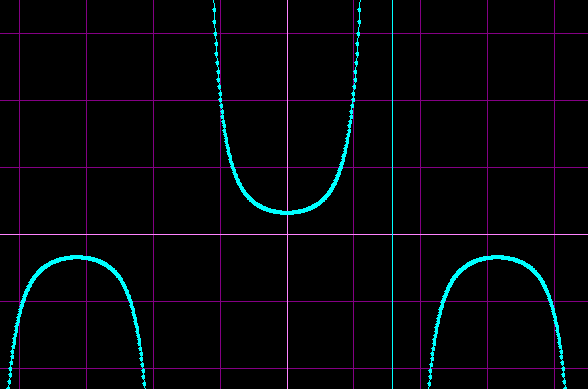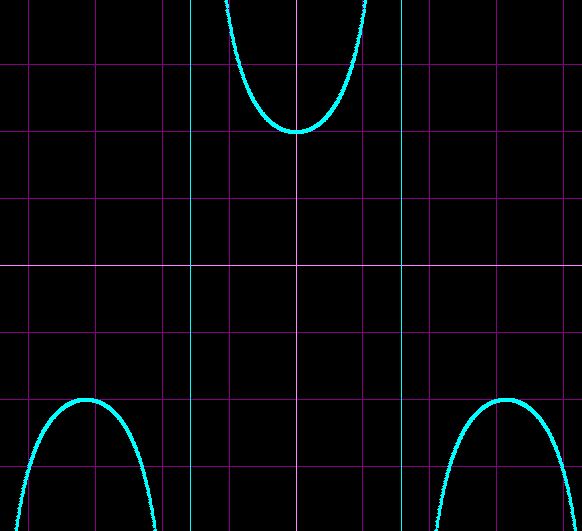# More substitution

• tony873004

Gold Member

## Homework Statement

$$\int {\sec ^3 x\,\,\tan x\,\,dx}$$

## Homework Equations

$$u = \sec x$$
This is my guess at u.

## The Attempt at a Solution

$$\frac{{du}}{{dx}} = \sec x\,\,\tan x,\,\,\,dx = \frac{{du}}{{\sec x\,\,\tan x}}$$

$$\int {\sec ^3 x\,\,\tan x\,\,dx} = \int {u^3 \,\,\tan x\,\,\frac{{du}}{{\sec x\,\,\tan x}}} = \int {u^3 \,\,\tan x\,\,\frac{{du}}{{u\,\,\tan x}} = }$$

$$\int {u^3 \,\,\,\,\frac{{du}}{u}} = \int {\frac{{u^3 }}{u}} du = \int {u^2 du} = 2u + C =$$

$$2\sec x + C$$

But the back of the book says
$$\frac{1}{3}\sec ^3 x + C$$

Playing around with it on the graphing software, I find that my answer and the book's answer produce different graphs, however, they both seem to have the problem stated in the question as their derivatives. Is one answer more correct than the other?

$$\frac{1}{3}\sec ^3 x + C$$$$2\sec x + C$$The integral of u2 is u3/3, not 2u. You differentiated instead of integrating.

oops. Thanks for catching that.

If the derivatives are equal, the functions can only differ by a constant. Do not accept answers as 'more correct', its right or wrong.

Edit: I just checked the post again, your working is a lot more than I'd have thought >.< Remember how substitution should be used when the function and its derivative is there? Rewrite the integral as:

$$\int \sec^2 x \sec x \tan x dx$$ Then Letting u= sec x, $$\int u^2 du$$, which seems faster to me ...just a tip.

Last edited:
Thanks, Gib. I checked the derivatives with the graphing software, dragging the derivative bar across the function, and watching it tangentally ride the curves of the function. But double checking this after fixing my error, I realized that the derivatives are almost, but not quite identical.

Thanks for the shorter method. We both got to u^2 du, but it took me more steps.